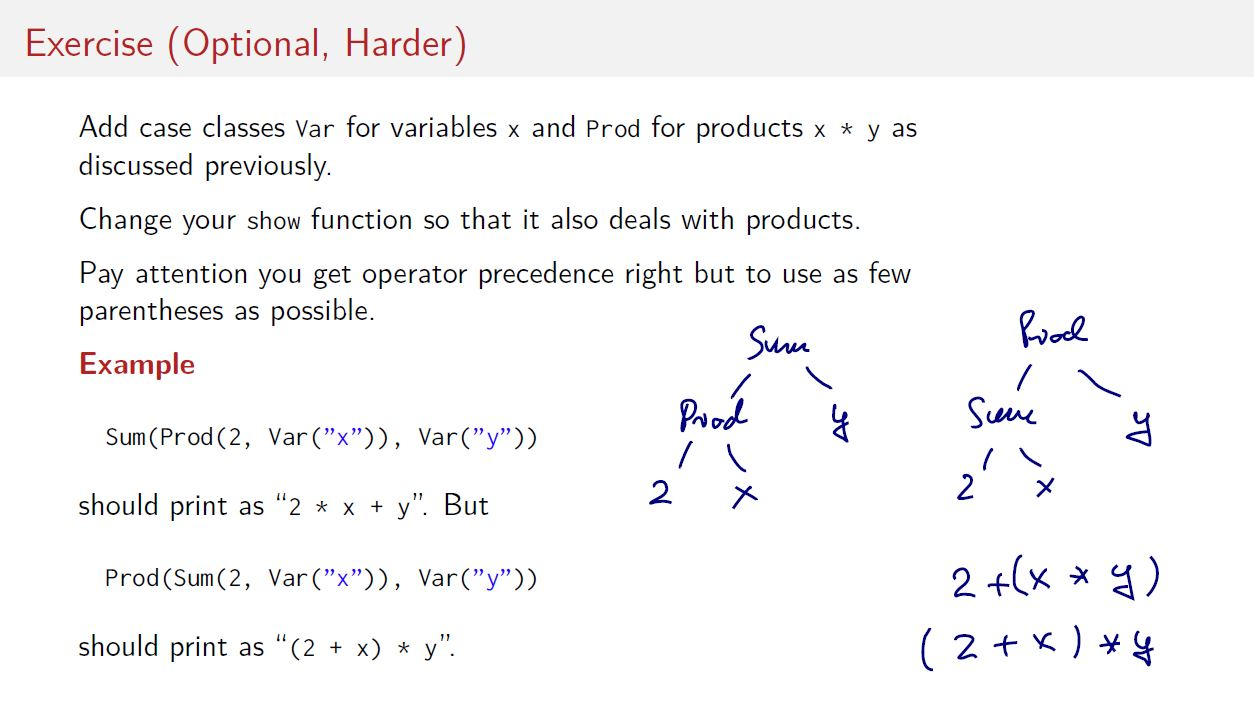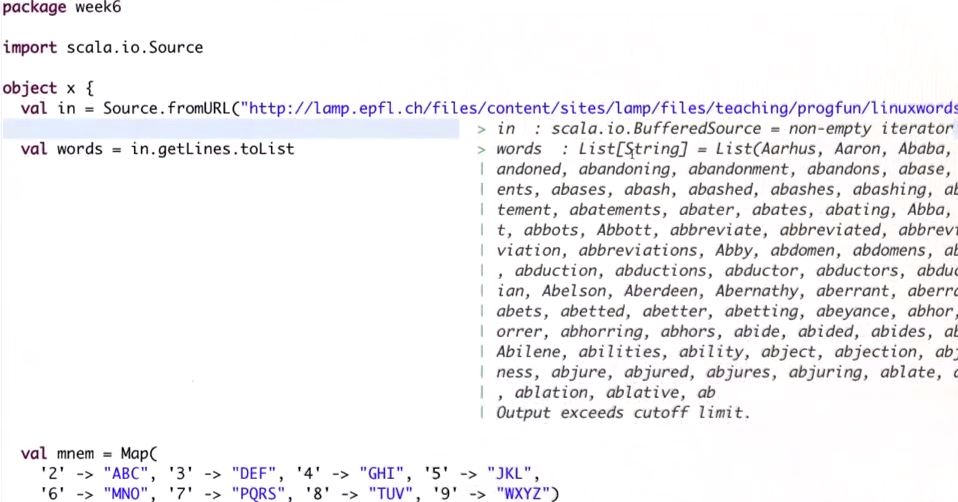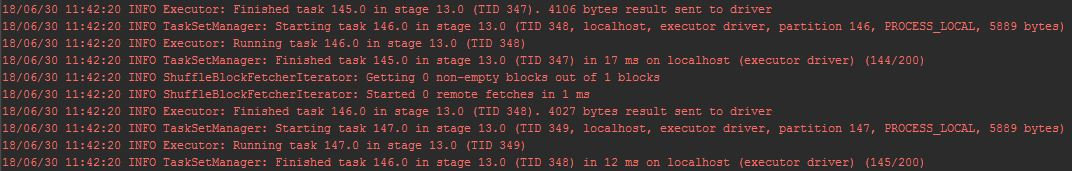# Functional Programming in Scala Specialization

## Review

Functional programming in scala specialization - Martin Odersky

### Info

This is an excellent course on mastering Scala, delivered in Coursera by the founder of the language himself, Prof. Martin Odersky from the famed École Polytechnique Fédérale de Lausanne (EPFL), Switzerland. Crucial parallel programming concepts, applicable in all programming languages, such as threads, parallel tasks, data parallelism etc., are taught by equally excellent teachers named Aleksandar Prokopec and Viktor Kuncak. Dr. Heather Miller covers Spark (distributed programming) concepts comprehensively including cluster topology, latency, transformation & actions, pair RDD, partitions, Spark SQL, Dataframes, etc. The teaching is accompanied with relevant hands-on exercises and coding assignments.

### Syllabus

This is a 4 course specialisation.

1. Functional Programming Principles in Scala
3. Parallel programming
4. Big Data Analysis with Scala and Spark

### Repository

The repository consists of the following:

• In-class exercises - codes (.scala and .sc worksheets)
• Projects - codes (.scala and .sc worksheets)

All the handouts and exercises of the course are also made available at EPFL’s Alaska website.

### Verified Certificate

1. IntelliJ-Idea setup
Users of IntelliJ-Idea as their Scala IDE might have encountered the following problem. Assume you have a scala class with accompanying methods written in a Foo.scala file.
 //File : Foo.scala
class Foo {
def foo1: Int = 2
}


Then you try to import the Foo class into a worksheet named Bar.sc to test the behaviour of your Foo class.

 //File : Bar.sc
import Foo
object Bar {
val bar1 = new Foo
}


Everything works fine initially. However, if you return to Foo.scala file and make some changes, these changes will not be picked up by your Bar.sc worksheet. Errors such as “Object cannot be found” (i.e., referring to your new/modified object in Foo.scala file) will be thrown when trying to run your worksheet. Rebuilding, recompiling, or “Make Project” does not help.

However, upon restarting the IntelliJ application, your Bar.sc worksheet will work flawlessly and detect the new changes in Foo.scala file.

Do the following to solve the problem:

• Go to File -> Settings -> Languages & Frameworks -> Scala -> Worksheet (tab)
• Unselect “Run worksheet in the compiler process”
2. Running tests in sbt
To test only specific classes (e.g., MySuite) and sepcific test cases (e.g., testName) in your test suite, execute the following command in sbt
 test:testOnly *MySuite -- -z testName


to run only the tests whose name includes the substring “testName”. For exact match rather than substring, use -t instead of -z.

## Course 1: Functional programming principles in Scala

### Week 4: Pattern matching

In lecture 5, week 4, on the topic of pattern matching, we are given a programming exercise shown in the image below, for which the solution was not given in class.I have provided my solution to this in-class exercise. The underscore _ acts as a wildcard representing anyting in Scala.

object week4_5{

trait Expr
case class Number(n: Int) extends Expr
case class Sum(e1: Expr, e2: Expr) extends Expr
case class Var(x: String) extends Expr
case class Prod(e1: Expr, e2: Expr) extends Expr

def show(e:Expr):String = {
def format(e:Expr):String = {
e match{
case Sum(_,_) => "(" + show(e) + ")"
case _ => show(e)
}
}
e match{
case Number(x) => x.toString
case Sum(l,r) => show(l) + "+" + show(r)
case Var(x) => x
case Prod(l,r) => format(l) + "*" + format(r)
}
}

show(Sum(Number(1),Number(64)))
show(Sum(Prod(Number(2),Var("x")),Var("y")))
show(Prod(Sum(Number(2),Var("x")),Var("y")))

}


The output upon evaluating the above worksheet is:

defined trait Expr
defined class Number
defined class Sum
defined class Var
defined class Prod

show: show[](val e: Expr) => String

res0: String = 1+64
res1: String = 2*x+y
res2: String = (2+x)*y


### Week 6: Putting the pieces together

In lecture 5, week 6, we are given a programming exercise on obtaining all phrases of words that can serve as mnemonics for a phone number. The URL to download the vocabulary is not clear from the instructional video.Hence, we provide the complete line of code to download the vocabulary:

val in = Source.fromURL("https://lamp.epfl.ch/files/content/sites/lamp/files/teaching/progfun/linuxwords.txt")


### Week 3 Assignment: ScalaCheck

In this week’s assignment, we are tasked with writing properties to be checked by ScalaCheck. The default assignment code guides us to write a heap generator genHeap of type Gen[H] and define an implicit val arbHeap of type Arbitrary[H]. When a property is executed, the forAll function searches for a corresponding implicit Arbitrary[H] generator matching its required input h:H. An example is given below to illustrate this, where the arbHeap will be used as the implicit generator to produce its input h of typ H.

  lazy val genHeap: Gen[H] = for {
x <- Arbitrary.arbitrary[A]
h <- Gen.frequency((45, Gen.const(empty)),(55,genHeap))
} yield insert(x, h)

implicit lazy val arbHeap: Arbitrary[H] = Arbitrary(genHeap)

property("property1") = forAll{(h: H) =>
val m = if (isEmpty(h)) 0 else findMin(h)
findMin(insert(m, h)) == m
}


In practice, it is best to avoid implicit parameters, such as implicit generators given by Arbitrary[T] values, to increase code comprehension. The above example is reproduced below with the generator for input h:H being explicitly defined as genHeap. This removes the need to define an intermediary implicit val.

  lazy val genHeap: Gen[H] = for {
x <- Arbitrary.arbitrary[A]
h <- Gen.frequency((45, Gen.const(empty)),(55,genHeap))
} yield insert(x, h)

property("property1") = forAll(genHeap){(h: H) =>
val m = if (isEmpty(h)) 0 else findMin(h)
findMin(insert(m, h)) == m
}


### Week 4 Lecture 3: Functional reactive programming

Odersky describes the “Signal” method for reactive programming, using a bank account example. In order to track the logic while using “Signal”, an “accounts.scala” file is provided in the repository (in addition to “accounts.sc” file) with many helpful print statements. Running “accounts.scala” file in debug mode, will print key values at intermediate stages, thus allowing us to track the logic behind “Signal” operation.

  println("xxx.caller->"+caller) //print identity of caller
println("xxx.observers->"+observers) //print identity of observers in the set
println("xxx.this->"+this) //print identitiy of the current object


In short, we should remember the following points to understand “Signal”:

1. On instantiation or upon being called, Signal A updates its formula/definition in the myExpr = () => expr variable. Signal A also updates its myValue variable.
2. Whenever an object B calls Signal A, Signal A stores the identity of the object B in its observers list. This is achieved via observers += caller.value in def apply() function.
3. Whenever a Signal A is updated or evaluated, it updates its myValue variable via myValue = newValue operation in protected def computeValue() function. Additionally, Signal A calls all of its observers to update their values too via observers = Set.empty; obs.foreach(_.computeValue()) in protected def computeValue() function.

Notice that the observers list of a Signal A is emptied after calling its observers. The observers list of Signal A will be populated again when some other object B calls Signal A in order to compute object B’s myValue variable.

### Week 4 Assignment: Calculator

Contrary to the Signal class introduced during Lecture 3, the Signal class provided in the assignment has an additional var observed besides the original var observers. Here observers refer to objects which are dependent on me whereas observed refers to objects that I depend on. The relevant portions of the code are shown below.

class Signal[T](expr: => T) {

private var observers: Set[Signal[_]] = Set()
private var observed: List[Signal[_]] = Nil
...

protected def computeValue(): Unit = {
for (sig <- observed)
sig.observers -= this
observed = Nil
...
}

...

def apply() = {
...
caller.value.observed ::= this
myValue
}
}


The principles of var observed is described next using an example. Assume we have 3 Signals defined as follows:

val a = Var(1)
val b = Var(a() + 1)
val c = Var(b() + 1)


On iniatilization of the three signals, the table below shows the var observers and var observed of each signal.

Signal observers observed
a b none
b c a
c none b

If at a later time, the expr of signal b is changed to b() = 2, then value of signal c will be recomputed accordingly. The var observers and var observed list will be updated to become:

Signal observers observed
a none none
b c none
c none b

Since signal b does not depend on signal a anymore, signal b is removed from var observers of signal a. Hence, in future, if signal a is updated, it does not need to inform or call signal b anymore.

Note that the Signal class works correctly even without var observed. In the example above, without use of var observed, signal a makes a redundant call to signal b when signal a is updated in the future. Essentially, use of var observed avoids unnecessary computations.

## Course 3: Parallel Programming

Scala codes used in the parallel programming lectures are available at the intructors GitHub website.

### Week 1 Lecture 3: Resolving Deadlocks

In this lecture, we are taught of class Account and of acquiring synchronized locks in the order of an unique ID to avoid deadlock situations. All instances of the class Account need to obtain an unique ID. This may be achieved via a companion object Account as shown next. Please refer to week1_2.sc worksheet in the repository for the complete working example using companion object.

object Account{
def apply(amount:Int = 0): Account = new Account(amount)

private var uidCount: Long = 0L

def getUniqueId():Long = this.synchronized {
uidCount = uidCount + 1
uidCount
}
}

class Account(private var amount: Int = 0){
import Account._

val uid = getUniqueId()

private def lockAndTransfer(target: Account, n:Int): Unit ={...}

def transfer(target: Account, n:Int): Unit = {...}
}


### Week 1 Lecture 8: Scalameter

In this week, we are introduced to Scalameter library to measure the performance of our Scala code in terms of time, memory, etc. Remember to use the right version of Scalameter library compatible to your installed Scala version. If in doubt, please verify the compatible versions at Maven Repository. Below are examples of compatible Scalameter libraries for different Scala versions.

scalaVersion := "2.11.7"
libraryDependencies += "com.storm-enroute" %% "scalameter-core" % "0.6"

scalaVersion := "2.12.4"
libraryDependencies += "com.storm-enroute" %% "scalameter-core" % "0.10"


## Course 4: Big Data Analysis with Scala and Spark

### Week 1 Lectures

1. While running Spark, your SBT shell may be inundated with INFO messages, preventing us from focusing on the program output.To suppress the above INFO messages of Spark, include the following in your Scala code:
 // Create spark session
val spark:SparkSession = SparkSession.builder()
.appName("MyApp")
.config("spark.master", "local")
.getOrCreate()
// Set log message level
spark.sparkContext.setLogLevel("ERROR")


### Week 2 Assignment: StackOverflow

A couple of key points on Option type and data shuffling is discussed below, which are useful in this week’s and general Spark programming.

1. Options can be viewed as collections. We can flatMap a Collection[Option[_]] to Collection[_]. For example we can flatMap List[Option[_]] to List[_]. For example, the following code
 object exercise {
// instantiate sample list
val num = List(1,2,3,4,5,6,7)
// method 1: map
val numMap = num.map(p => if (p%2 == 0) Some(p*2) else None)
// method 2: flatMap
val numFlatMap = num.flatMap(p => if (p%2 == 0) Some(p*2) else None)
// method 3: flatMap in for loop
for {
num: Option[Int] <- numMap
out: Int <- num
} yield out
}


will output:

 num: List[Int] = List(1, 2, 3, 4, 5, 6, 7)

numMap: List[Option[Int]] = List(None, Some(4), None, Some(8), None, Some(12), None)

numFlatMap: List[Int] = List(4, 8, 12)

res0: List[Int] = List(4, 8, 12)

2. For each iteration, in the k-means clustering, we need to group points together and update the cluster mean values. The first method below is straightforward, but it is slow due to large data shuffling since we use groupBy first.
 val cluster: RDD[(Int, Iterable[(LangIndex, HighScore)])] = vectors.groupBy(p => findClosest(p,means))
val newMeansRdd: Array[(Int, (LangIndex, HighScore))] = cluster.mapValues(p => averageVectors(p)).collect()


An alternative method provided below is faster, since we create a pair RDD and use reduceByKey first, thus reducing the amount of data shuffling. However, this method should not be used in this assignment since the averageVectors method is not associative due to the use of .toInt rounding function.

 val cluster: RDD[(Int, (LangIndex, HighScore))] = vectors.map(p => (findClosest(p,means),p))
val newMeansRdd: Array[(Int, (LangIndex, HighScore))] = cluster.reduceByKey((a, b) => averageVectors(Iterable(a,b))).collect()


### Week 4 Lectures: Dataframes

Some of the in-class Spark exercises are provided as .scala file in Big Data Analysis with Scala and Spark -> exercise folder of the repository, for the readers to try out and get an understanding of the Spark Dataframe APIs.

Additionally examples of Spark transformations and actions on Dataframes are also implemented in Databricks Community and the notebook is available in this course repository.

Some of the operations excuted in Databricks Community.

import org.apache.spark.sql.functions._
import org.apache.spark.sql.SparkSession

// Create spark session
val spark:SparkSession = SparkSession.builder()
.appName("MyApp")
.getOrCreate()

// Create spark context
import spark.implicits._

// Define dataset
case class Employee(id: Int, fname:String, lname:String, work:Array[Int], city:String, age:Int)
val emp = Seq(Employee(12,"Joe","Smith",Array(38,67,89),"New York",34),
Employee(645,"Slate","Markham",Array(28,3),"Sydney",2),
Employee(12,"Sally","Owens",Array(48,1,0),"New York",12),
Employee(221,"Joe","Walker",Array(21),"Sydney",89),
Employee(12,"Joe","Runner",Array(21),"Sydney",89),
Employee(645,"Slate","Ontario",Array(12,3),"Wellington",25))
// Convert dataset to RDD
val empRDD = spark.sparkContext.parallelize(emp)
// Convert dataset to Dataframe
val empDF = emp.toDF
// Convert Dataframe to RDD
val empRDDfromDF = empDF.rdd

// Visualize Dataframe
empDF.printSchema()
empDF.show()

root
|-- id: integer (nullable = false)
|-- fname: string (nullable = true)
|-- lname: string (nullable = true)
|-- work: array (nullable = true)
|    |-- element: integer (containsNull = false)
|-- city: string (nullable = true)
|-- age: integer (nullable = false)

+---+-----+-------+------------+----------+---+
| id|fname|  lname|        work|      city|age|
+---+-----+-------+------------+----------+---+
| 12|  Joe|  Smith|[38, 67, 89]|  New York| 34|
|645|Slate|Markham|     [28, 3]|    Sydney|  2|
| 12|Sally|  Owens|  [48, 1, 0]|  New York| 12|
|221|  Joe| Walker|        |    Sydney| 89|
| 12|  Joe| Runner|        |    Sydney| 89|
|645|Slate|Ontario|     [12, 3]|Wellington| 25|
+---+-----+-------+------------+----------+---+

val selectedemp = empDF.select($"id",$"lname")
.where($"city" === "Sydney") .orderBy($"id")
selectedemp.show()

val rankedemp = empDF.groupBy($"id") .max("age") rankedemp.show() val rank = empDF.groupBy($"id",$"fname") .agg(count($"id"))
.orderBy($"fname",$"count(id)".desc)
rank.show()

+---+-------+
| id|  lname|
+---+-------+
| 12| Runner|
|221| Walker|
|645|Markham|
+---+-------+

+---+--------+
| id|max(age)|
+---+--------+
| 12|      89|
|645|      25|
|221|      89|
+---+--------+

+---+-----+---------+
| id|fname|count(id)|
+---+-----+---------+
| 12|  Joe|        2|
|221|  Joe|        1|
| 12|Sally|        1|
|645|Slate|        2|
+---+-----+---------+